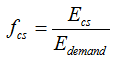HOMER Pro 3.13

 Navigation: Glossary Capacity Shortage FractionType: Output Variable Units: none Symbol: fcs

The capacity shortage fraction is equal to the total capacity shortage divided by the total electrical demand. HOMER considers a system feasible (or acceptable) only if the capacity shortage fraction is less than or equal to the maximum annual capacity shortage. HOMER uses the following equation to calculate the capacity shortage fraction:where: Ecs = total capacity shortage [kWh/yr] Edemand = total electrical demand (primary and deferrable load) [kWh/yr]